# Problem Solving Question: Word Problem - Rates

This one is a word problem in Rates - Work and Time. Word problems test your ability to translate information given in words into mathematical expressions and equations and solve them. The gist of what is tested is the ability to translate effectively.

## Question

• PS
• Working alone at their respective constant rates, A can complete a task in ‘a’ days and B in ‘b’ days. They take turns in doing the task with each working 2 days at a time. If A starts they finish the task in exactly 10 days. If B starts, they take half a day more. How long does it take to complete the task if they both work together? A.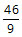B.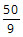C.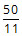D.E.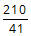Choice D. They will complete the task indays

Click to access Explanatory Answer slide deck

## Detailed Solution

Understanding relation between rate and time
Working alone at a constant rate if A takes 'a' days to complete a task, A will complete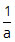of the task in a day.

## Example 1

For e.g, If A takes 20 days to complete a task and B takes 30 days to complete a task, it will be INCORRECT to frame an equation a + b = 50

The correct way to express this information is:
A will complete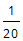th of the task in a day.
B will complete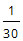th of the task in a day.
Working together they will complete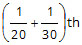of the task in day.
i.e., they will completeof the task in day.
So, they will complete the task in 12 days.

## Example 2

Let us look at another example.

A takes 'a' days to complete a task and B takes 'b' days to complete the same task. If they work together they complete the task in 10 days.

It is INCORRECT to frame the equation as a + b = 10.

The correct expression isTranslate words into mathematical expressions

## They take turns in doing the task with each working 2 days at a time.

A will completeof the task in a day.

Therefore, in 2 days A will complete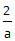of the task in a day.

Similarly, A will complete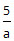of the task in 5 days.

## If A starts they finish the task in exactly 10 days.

A starts and works for 2 days. So, A will work on day 1 and day 2.
Then B will work for the next 2 days. B will work on day 3 and day 4.
A will continue for the next 2 days. i.e., on day 5 and day 6.
B will work on day 7 and day 8.
A will for the last 2 days i.e., day 9 and day 10.

Therefore, A will work on day 1, day 2, day 5, day 6, day 9, and day 10. i.e., for 6 days.
And B will work on day 3, day 4, day 7, and day 8. i.e., for 4 days.

In 6 days A will complete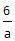of the task.
In 4 days B will complete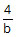of the task.

With A working 6 days and B working 4 days, the task is completed.
i.e.,+= 1 .... eqn (1)

## If B starts they finish the task they take half a day more. i.e., 10.5 days.

Therefore, B will work on day 1, day 2, day 5, day 6, day 9, and day 10. i.e., for 6 days.
And A will work on day 3, day 4, day 7, day 8 and half a day on day 11. i.e., for 4.5 days.

In 6 days B will complete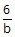of the task.
In 4.5 days A will complete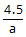of the task.

With B working 6 days and A working 4.5 days, the task is completed.
i.e.,+= 1 .... eqn (2)

Solve the two equations+= 1 .... eqn (1)+= 1 .... eqn (2)

Solving the two equations we get a = 9 days and b = 12 days.

## The question: How long does it take to complete the task if they both work together?

Working together A and B will complete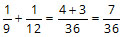th of the task in a day.

Hence, they will complete the task indays.